Home Practice
For learners and parents For teachers and schools
Textbooks
Full catalogue
Pricing SupportLog in

We think you are located in United States. Is this correct?

# Chapter 11: Trigonometry

• This chapter covers solving problems in two-dimensions using trigonometry.
• Emphasise the value and importance of making sketches, where appropriate.
• Prior to starting this chapter it may be appropriate to quickly revise the earlier content on trigonometry.

Trigonometry was developed in ancient civilisations to solve practical problems such as building construction and navigating by the stars. We will show that trigonometry can also be used to solve some other practical problems. We can use the trigonometric ratios to solve problems in two dimensions that involve right-angled triangles.

As revision the three trigonometric ratios can be defined for right-angled triangles as:

\begin{align*} \sin \theta & = \frac{\text{opposite}}{\text{hypotenuse}} \\ \cos \theta & = \frac{\text{adjacent}}{\text{hypotenuse}} \\ \tan \theta & = \frac{\text{opposite}}{\text{adjacent}} \end{align*}

We will use these three ratios and the theorem of Pythagoras to help us solve two-dimensional problems.

## 11.1 Two-dimensional problems (EMA7F)

In two-dimensional problems we will often refer to the angle of elevation and the angle of depression. To understand these two angles let us consider a person sailing alongside some cliffs. The person looks up and sees the top of the cliffs as shown below: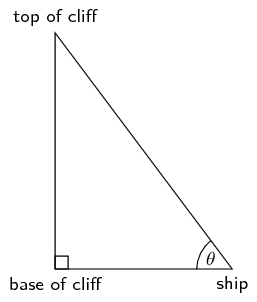In this diagram $$\theta$$ is the angle of elevation.

Angle of elevation
The angle of elevation is the angle formed by the line of sight and the horizontal plane for an object above the horizontal plane.

In our diagram the line of sight is from the ship to the top of the cliffs. The horizontal plane is from the ship to the base of the cliffs. Also note the we can consider the cliffs to be a straight vertical line and so we have a right-angled triangle.

To understand the angle of depression let us now consider the same situation as above but instead our observer is standing on top of the cliffs looking down at the ship.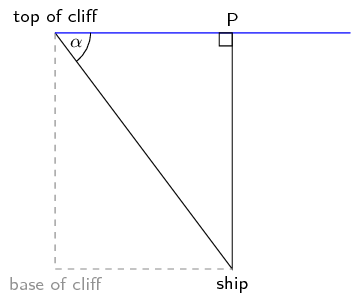In this diagram $$\alpha$$ is the angle of depression.

Angle of depression
The angle of depression is the angle formed by the line of sight and the horizontal plane for an object below the horizontal plane.

In our diagram the line of sight is from the top of the cliffs to the ship. The horizontal plane is from the top of the cliffs through $$P$$. Note that this is parallel to the line between the base of the cliffs and the ship. $$P$$ lies directly above the ship. We can construct a vertical, perpendicular line to the horizontal plane at the point $$P$$.

Finally we can compare the angle of elevation and the angle of depression. In the following diagram the line from the base of the cliffs to the ship is parallel to the line from the top of the cliffs to $$P$$. The angle of elevation and the angle of depression are indicated. Notice that $$\alpha = \theta$$.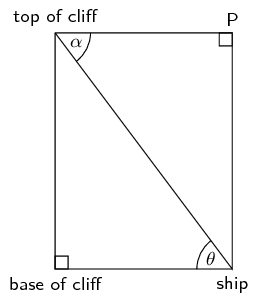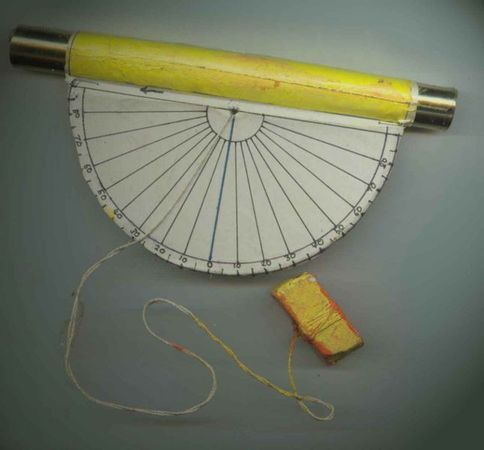An inclinometer. Inclinometers can be used to measure angles of inclination and so can be used to determine the height of an object.

You can make an inclinometer to measure the angle of inclination of a tall building or tree. Once you have the angle of inclination you can determine the height of the building or tree.

In trigonometry the angle of inclination is the same as the angle of elevation.

temp text

## Worked example 1: Flying a kite

Mandla flies a kite on a $$\text{17}$$ $$\text{m}$$ string at an inclination of $$\text{63}$$°.

1. What is the height, $$h$$, of the kite above the ground?

2. If Mandla's friend Sipho stands directly below the kite, calculate the distance, $$d$$, between the two friends.

### Make a sketch and identify the opposite and adjacent sides and the hypotenuse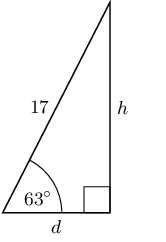### Use given information and appropriate ratio to solve for $$h$$ and $$d$$

1. \begin{align*} \sin 63° & = \frac{\text{opposite}}{\text{hypotenuse}} \\ \sin 63° & = \frac{h}{17} \\ \therefore h & = 17 \sin 63° \\ & = \text{15,14711...} \\ & \approx \text{15,15}\text{ m} \end{align*}
2. \begin{align*} \cos 63° & = \frac{\text{adjacent}}{\text{hypotenuse}} \\ \cos 63° & = \frac{d}{17} \\ \therefore d & = 17 \cos 63° \\ & = \text{7,7178...} \\ & \approx \text{7,72}\text{ m} \end{align*}

Note that the third side of the triangle can also be calculated using the theorem of Pythagoras: $${d}^{2} = {17}^{2} - {h}^{2}$$.

1. The kite is $$\text{15,15}$$ $$\text{m}$$ above the ground.

2. Mandla and Sipho are $$\text{7,72}$$ $$\text{m}$$ apart.

## Worked example 2: Calculating angles

$$ABCD$$ is a trapezium with $$AB=\text{4}\text{ cm}$$, $$CD=\text{6}\text{ cm}$$, $$BC=\text{5}\text{ cm}$$ and $$AD=\text{5}\text{ cm}$$. Point $$E$$ on diagonal $$AC$$ divides the diagonal such that $$AE=\text{3}\text{ cm}$$. $$B\hat{E}C = 90°$$. Find $$A\hat{B}C$$.

### Draw trapezium and label all given lengths on diagram. Indicate that $$B\hat{E}C = 90°$$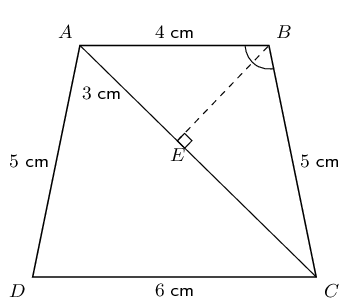We will use $$\triangle ABE$$ and $$\triangle CBE$$ to find $$A\hat{B}E$$ and $$C\hat{B}E$$. We can then add these two angles together to find $$A\hat{B}C$$.

### Find the first angle, $$A\hat{B}E$$

The hypotenuse and opposite side are given for both triangles, therefore use the $$\sin$$ function.

In $$\triangle ABE$$:

\begin{align*} \sin A\hat{B}E & = \frac{\text{opposite}}{\text{hypotenuse}} \\ & = \frac{3}{4} \\ A\hat{B}E & = \text{48,5903...} \\ & \approx \text{48,6}° \end{align*}

### Use the theorem of Pythagoras to determine $$BE$$

In $$\triangle ABE$$:

\begin{align*} BE^{2} & = AB^{2} - AE^{2} \\ & = {4}^{2} - {3}^{2} \\ & = 7 \\ \therefore BE & = \sqrt{7}\text{cm} \end{align*}

### Find the second angle $$C\hat{B}E$$

In $$\triangle CBE$$:

\begin{align*} \cos C\hat{B}E & = \frac{\text{adjacent}}{\text{hypotenuse}} \\ & = \frac{\sqrt{7}}{5} \\ & = \text{0,52915...} \\ C\hat{B}E & = \text{58,0519...} \\ & \approx \text{58,1}° \end{align*}

### Calculate the sum of the angles

$A\hat{B}C = \text{48,6}° + \text{58,1}° = \text{106,7}°$

Another application is using trigonometry to find the height of a building. We could use a tape measure lowered from the roof, but this is impractical (and dangerous) for tall buildings. It is much more sensible to use trigonometry.

## Worked example 3: Finding the height of a building

The given diagram shows a building of unknown height $$h$$. We start at point $$B$$ and walk $$\text{100}$$ $$\text{m}$$ away from the building to point $$Q$$. Next we measure the angle of elevation from the ground to the top of the building, $$T$$, and find that the angle is $$\text{38,7}°$$. Calculate the height of the building, correct to the nearest metre.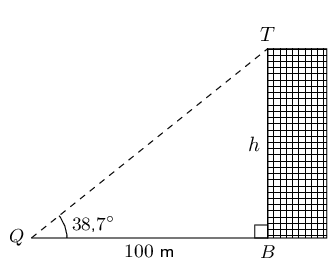### Identify the opposite and adjacent sides and the hypotenuse

We have a right-angled triangle and know the length of one side and an angle. We can therefore calculate the height of the building.

### Rearrange and solve for $$h$$

\begin{align*} h & = 100 \times \tan \text{38,7}° \\ & = \text{80,1151...} \\ & \approx \text{80} \end{align*}

The height of the building is $$\text{80}$$ $$\text{m}$$.

## Worked example 4: Angles of elevation and depression

A block of flats is $$\text{200}$$ $$\text{m}$$ away from a cellphone tower. Someone stands at $$B$$. They measure the angle from $$B$$ to the top of the tower ($$E$$) to be $$\text{34}$$$$\text{°}$$ (the angle of elevation). They then measure the angle from $$B$$ to the bottom of the tower ($$C$$) to be $$\text{62}$$$$\text{°}$$ (the angle of depression).

What is the height of the cellphone tower (correct to the nearest metre)?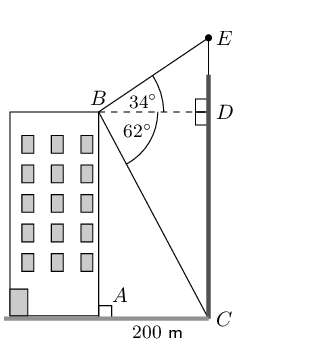Note: diagram not drawn to scale

### To determine height $$CE$$, first calculate lengths $$DE$$ and $$CD$$

$$\triangle BDE$$ and $$\triangle BDC$$ are both right-angled triangles. In each of the triangles, the length $$BD$$ is known. Therefore we can calculate the sides of the triangles.

### Calculate $$CD$$

The length $$AC$$ is given. $$CABD$$ is a rectangle so $$BD = AC = \text{200}\text{ m}$$.

In $$\triangle CBD$$:

\begin{align*} \tan C\hat{B}D & = \frac{CD}{BD} \\ \therefore CD & = BD \times \tan C\hat{B}D \\ & = 200 \times \tan 62° \\ & = \text{376,1452...} \\ & \approx \text{376}\text{ m} \end{align*}

### Calculate $$DE$$

In $$\triangle DBE$$:

\begin{align*} \tan D\hat{B}E & = \frac{DE}{BD} \\ \therefore DE & = BD \times \tan D\hat{B}E \\ & = 200 \times \tan 34° \\ & = \text{134,9017...} \\ & \approx \text{135}\text{ m} \end{align*}

The height of the tower is: $$CE = CD + DE = \text{135}\text{ m} + \text{376}\text{ m} = \text{511}\text{ m}$$.

temp text

## Worked example 5: Building plan

Mr Nkosi has a garage at his house and he decides to add a corrugated iron roof to the side of the garage. The garage is $$\text{4}$$ $$\text{m}$$ high, and his sheet for the roof is $$\text{5}$$ $$\text{m}$$ long. If the angle of the roof is $$\text{5}$$°, how high must he build the wall $$BD$$? Give the answer correct to $$\text{1}$$ decimal place.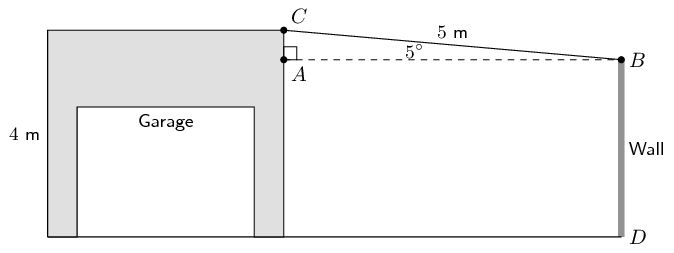### Identify opposite and adjacent sides and hypotenuse

$$\triangle ABC$$ is right-angled. The hypotenuse and an angle are known therefore we can calculate $$AC$$. The height of the wall $$BD$$ is then the height of the garage minus $$AC$$.

\begin{align*} \sin A\hat{B}C & = \frac{AC}{BC} \\ \therefore AC & = BC \times \sin A\hat{B}C \\ & = 5 \sin 5° \\ & = \text{0,43577...} \\ & \approx \text{0,4}\text{ m} \\ \\ \therefore BD& = \text{4}\text{ m} - \text{0,4}\text{ m} \\ & = \text{3,6}\text{ m} \end{align*}

Mr Nkosi must build his wall to be $$\text{3,6}$$ $$\text{m}$$ high.

Textbook Exercise 11.1

A person stands at point $$A$$, looking up at a bird sitting on the top of a building, point $$B$$.

The height of the building is $$x$$ meters, the line of sight distance from point $$A$$ to the top of the building (point $$B$$) is $$\text{5,32}$$ meters, and the angle of elevation to the top of the building is $$70^\circ$$.

Calculate the height of the building ($$x$$) as shown in the diagram below: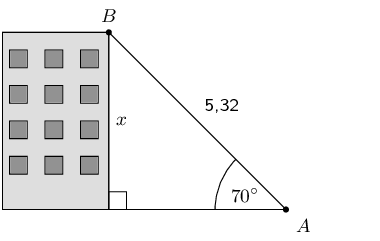\begin{align*} \sin \theta & = \frac{\text{opposite}}{\text{hypotenuse}}\\ \sin \text{70}^\circ & = \frac{x}{\text{5,32}} \\ x & = \text{4,99916...} \\ & \approx \text{5} \end{align*}

The height of the building is $$\text{5}$$ $$\text{m}$$.

A person stands at point $$A$$, looking up at a bird sitting on the top of a pole (point $$B$$).

The height of the pole is $$x$$ meters, point A is $$\text{4,2}$$ meters away from the foot of the pole, and the angle of elevation to the top of the pole is $$65^\circ$$.

Calculate the height of the pole ($$x$$), to the nearest metre.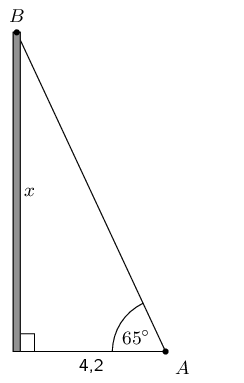\begin{align*} \tan{\theta} & = \frac{\text{opposite}}{\text{adjacent}}\\ \tan{\text{65}^\circ} & = \frac{x}{\text{4,2}} \\ x & = \text{9,0069...} \\ & \approx \text{9} \end{align*}

The height of the pole is $$\text{9}$$ $$\text{m}$$.

A boy flying a kite is standing $$\text{30}$$ $$\text{m}$$ from a point directly under the kite. If the kite's string is $$\text{50}$$ $$\text{m}$$ long, find the angle of elevation of the kite.

First draw a sketch: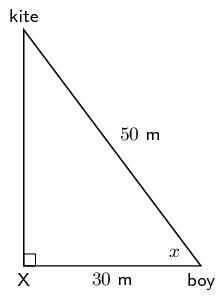We can use the cosine ratio to find the angle of elevation ($$x$$):

\begin{align*} \cos x & = \frac{30}{50} \\ x & = \text{53,1301...} \\ & \approx \text{53,13}° \end{align*}

The angle of elevation of the kite is $$\text{53,13}$$°.

What is the angle of elevation of the sun when a tree $$\text{7,15}$$ $$\text{m}$$ tall casts a shadow $$\text{10,1}$$ $$\text{m}$$ long?

First draw a sketch: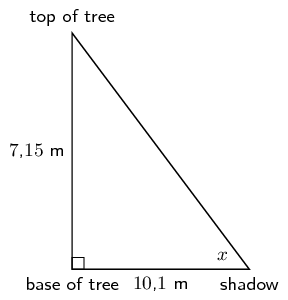We can use the tangent ratio to find the angle of elevation ($$x$$):

\begin{align*} \tan x & = \frac{\text{7,15}}{\text{10,1}} \\ x & = \text{35,2954...} \\ & \approx \text{35,30}° \end{align*}

The angle of elevation of the sun is $$\text{35,30}$$°.

From a distance of $$\text{300}$$ $$\text{m}$$, Susan looks up at the top of a lighthouse. The angle of elevation is $$\text{5}$$°. Determine the height of the lighthouse to the nearest metre.

First draw a sketch: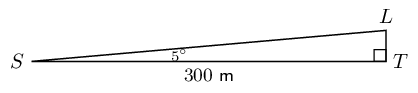We need to find $$LT$$. We can use the tangent ratio:

\begin{align*} \tan \hat{S} & = \frac{LT}{ST} \\ LT & = 300 \tan 5° \\ & = \text{26,2465...} \\ & \approx \text{26}\text{ m} \end{align*}

The height of the lighthouse is $$\text{26}$$ $$\text{m}$$.

A ladder of length $$\text{25}$$ $$\text{m}$$ is resting against a wall, the ladder makes an angle $$\text{37}$$° to the wall. Find the distance between the wall and the base of the ladder to the nearest metre.

First draw a sketch: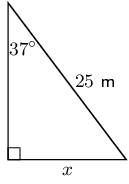Notice that we are given the angle that the ladder makes with the wall, not the angle that the ladder makes with the ground.

Now we can use the sine function to find $$x$$:

\begin{align*} \sin 37° & = \frac{x}{25} \\ x & = 25 \sin 37° \\ & = \text{15,04537...} \\ & \approx \text{15}\text{ m} \end{align*}

The base of the ladder is $$\text{15}$$ $$\text{m}$$ away from the wall.